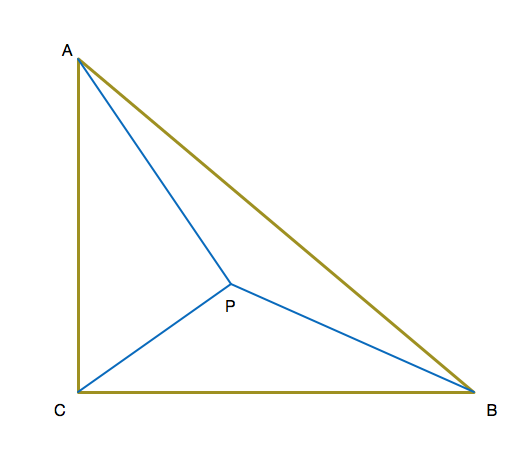# Such Triangle

Geometry Level 5Isosceles triangle ABC has a right angle at C. Point P is somewhere inside ABC such that PA=11, PB=7, and PC=6. Legs AC and BC have length s= $\sqrt{a+b\sqrt2}$. Find a+b.

• This problem is taken from a reviewer given to me. I take no credit.
×